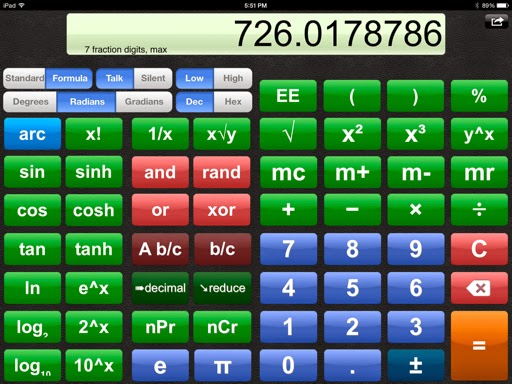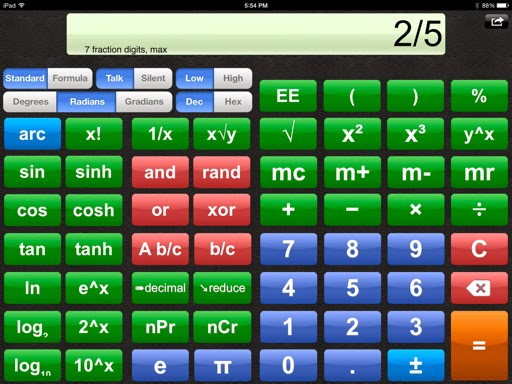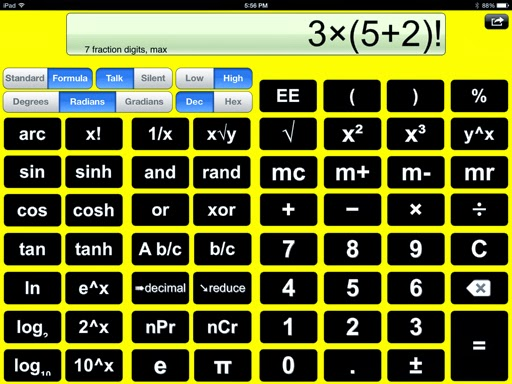## Tuesday, April 28, 2015

### HP Prime: Mechanical Engineering Programs by Djajadi Susastra

Hello everyone, still on vacation but I'm back in the Los Angeles area.

I received an email from retired engineer Djajadi Susastra, who has been using Hewlett Packard calculators since 1989.  Susastra obtained a BSc in Mechanical Engineering that year.  He recently purchased an HP Prime calculator and has asked me to share his programs on mechanical engineering (the last three deal with business and finance), which he learned how to program the Prime from this blog.

Djajadi, I am very thankful for the compliments and with pleasure, here are the programs he has sent:

CALCULATING CROSS SECTION AREA OF CYLINDER.

EXPORT AREA()
BEGIN
LOCAL OD, ID, AA;
INPUT (OD, "OD=" );
INPUT (ID,"ID=" );
AA:=(OD^2-ID^2)/4*PI;
MSGBOX ("XSECTION AREA=" +AA);

END;

CALCULATING MAXIMUM BENDING MOMENT ON A CYLINDER AT YIELD.

EXPORT BENDING()
BEGIN
LOCAL OD, ID, YI, I, BEN;
INPUT (OD, "OD (IN)=" );
INPUT (ID, "ID= (IN)=" );
INPUT (YI, "YIELD (PSI)=");
I:=(OD^4-ID^4)/64*PI;
BEN:=YI*I/(12*(OD/2));
MSGBOX ("MAX BENDING ALLOWED (FT-LB)=" +BEN);

END;

CALCULATING COLLAPSE PRESSURE ON 110 KSI YIELD PIPE (PER API 5C3).

EXPORT COLLAPSE110()
BEGIN
LOCAL OD, ID, YI, TEN, DT, COL;
INPUT (OD, "OD (IN)=" );
INPUT (ID, "ID= (IN)=" );
YI:=110000;

DT:=OD/((OD-ID)/2);

IF DT≤12.44 THEN
COL:=2*YI*(DT-1)/DT^2;
MSGBOX ("COLLAPSE PRESS (PSI)=" +COL);
END;

IF 12.44<DT AND DT≤20.41 THEN
COL:=YI*(3.181/DT-.0819)-2852;
MSGBOX ("COLLPASE PRESS (PSI)=" +COL);
END;

IF 20.41<DT AND DT≤26.22 THEN
COL:=YI*(2.066/DT-.0532);
MSGBOX ("COLLAPSE PRESS (PSI)=" +COL);
END;

IF 26.22<DT AND DT≤1000 THEN
COL:=46950000/(DT*(DT-1)^2);
MSGBOX ("COLLAPSE PRESS (PSI)=" +COL);
END;

IF DT≥1001 THEN
MSGBOX ("NO CASE");

END;
END;

CALCULATING COLLAPSE PRESSURE ON 80 KSI YIELD PIPE (PER API 5C3).

EXPORT COLLAPSE80()
BEGIN
LOCAL OD, ID, YI, TEN, DT, COL;
INPUT (OD, "OD (IN)=" );
INPUT (ID, "ID= (IN)=" );
YI:=80000;

DT:=OD/((OD-ID)/2);

IF DT≤13.38 THEN
COL:=2*YI*(DT-1)/DT^2;
MSGBOX ("COLLAPSE PRESS (PSI)=" +COL);
END;

IF 13.38<DT AND DT≤22.47 THEN
COL:=YI*(3.071/DT-.0667)-1955;
MSGBOX ("COLLPASE PRESS (PSI)=" +COL);
END;

IF 22.47<DT AND DT≤31.02 THEN
COL:=YI*(1.998/DT-.0434);
MSGBOX ("COLLAPSE PRESS (PSI)=" +COL);
END;

IF 31.02<DT AND DT≤1000 THEN
COL:=46950000/(DT*(DT-1)^2);
MSGBOX ("COLLAPSE PRESS (PSI)=" +COL);
END;

IF DT≥1001 THEN
MSGBOX ("NO CASE");

END;
END;

CALCULATING COLLAPSE PRESSURE ON 75KSI YIELD PIPE (PER API 5C3).

EXPORT COLLAPSE75()
BEGIN
LOCAL OD, ID, YI, TEN, DT, COL;
INPUT (OD, "OD (IN)=" );
INPUT (ID, "ID= (IN)=" );
YI:=75000;

DT:=OD/((OD-ID)/2);

IF DT≤13.6 THEN
COL:=2*YI*(DT-1)/DT^2;
MSGBOX ("COLLAPSE PRESS (PSI)=" +COL);
END;

IF 13.6<DT AND DT≤22.91 THEN
COL:=YI*(3.054/DT-.0642)-1806;
MSGBOX ("COLLPASE PRESS (PSI)=" +COL);
END;

IF 22.91<DT AND DT≤32.05 THEN
COL:=YI*(1.99/DT-.0418);
MSGBOX ("COLLAPSE PRESS (PSI)=" +COL);
END;

IF 32.05<DT AND DT≤1000 THEN
COL:=46950000/(DT*(DT-1)^2);
MSGBOX ("COLLAPSE PRESS (PSI)=" +COL);
END;

IF DT≥1001 THEN
MSGBOX ("NO CASE");

END;
END;

CALCULATING VON MISSES STRESS (THICK WALL, SHIGLEY AND MITCHELL, API 6A)

EXPORT EQUI_STRESS()
BEGIN
LOCAL OD, ID, P, S1, S2, S3, SE, T;

INPUT (OD, "OD (IN)=" );
INPUT (ID, "ID= (IN)=" );
INPUT (P, "PRESSURE (PSI)=");

T:=(OD-ID)/2;
LOCAL RA;
RA:=OD/40;

IF T>RA THEN
S1:=P*ID^2/(OD^2-ID^2);
S2:=P*(OD^2+ID^2)/(OD^2-ID^2);
S3:=-1*P;
SE:=(((S1-S2)^2+(S2-S3)^2+(S3-S1)^2)/2)^.5;
MSGBOX ("EQUIVALENT STRESS (PSI)=" +SE);
ELSE
MSGBOX ("THIN WALL");
END;
END;

CALCULATING YIELD PRESSURE (API 5 C3).

EXPORT YIELDPRESS()
BEGIN
LOCAL OD, ID, YI, PY;
INPUT (OD, "OD (IN)=" );
INPUT (ID, "ID= (IN)=" );
INPUT (YI, "YIELD (PSI)=");
PY:=.875*(2*YI*(OD-ID)/2)/OD;
MSGBOX ("YIELD PRESSURE (PSI)=" +PY);
RETURN
END;

CALCULATING SHEAR AREA AND BEARING AREA ON ACME THREADS.

BEGIN
LOCAL D, N, P, ES, KN, M, SA, BA;
INPUT (D, "OD=" );
INPUT (N,"NO OF THREAD PER INCH" );
P:=1/N;
ES:=D-.5*P-.008*D^.5-.006*D^.5-.03*(1/N)^.5;
KN:=D-P+.05*P;
M:=D-.05*P;
SA:=PI*KN*(.5+N*TAN(14.5)*(ES-KN));
BA:=(M^2-KN^2)/4*N*PI;
MSGBOX ("ACME 2G, SHEAR AREA=" +SA);
MSGBOX ("BEARING AREA=" +BA);

END;

CALCULATING GROSS MARGIN (IN %) WHEN COST AND PRICE ARE KNOWN.

EXPORT COST()
BEGIN
LOCAL P, C, GM;
INPUT (P, "PRICE=" );
INPUT (C, "COST=" );
GM:=100-(100*C/P);
MSGBOX ("GROSS MARGIN (%)=" +GM);

END;

CALCULATING PRICE WHEN COST AND GROSS MARGIN (IN %) ARE KNOWN.

EXPORT GROSSMARGIN()
BEGIN
LOCAL G, C, P;
INPUT (G, "GM (%)" );
INPUT (C, "COST=" );
P:=C/(1-G/100);
MSGBOX ("PRICE=" +P);
RETURN
END;

CALCULATING FUTURE VALUE WHEN PRESENT VALUE, INTEREST PER YEAR, AND PERIOD IN YEAR ARE KNOWN.

EXPORT FUTUREVAL ()
BEGIN
LOCAL PV, I, P, N, FV;
INPUT (PV, "PRESENT VALUE=" );
INPUT (I,"INTEREST (ANNUAL)=" );
INPUT (N, "PERIOD (IN YEAR)=");
FV:=PV*(1+I/100)^N;
MSGBOX ("FUTURE VALUE=" +FV);

END

Eddie

This blog entry is property of Edward Shore.  2015.

## Thursday, April 23, 2015

### HP Prime and TI-84+: Rotation and Translation of the 3-D Vector

HP Prime and TI-84+:  Rotation and Translation of the 3-D Vector

Greetings from Monterey, California!Greetings from Cal State Monterey BayDeep Sea Tank:  Monterey Bay Aquarium

Let P be a three dimensional column vector P = [[ x ],[ y ],[ z ]].

We can move P by a linear transformation by addition to get a new vector P’:

P’ = P + T where T = [[ t1 ],[ t2 ],[ t3 ]].

We can rotate point P by using one of three rotation matrices using left-multiplication:

P’ = RP  where R can take the form:

Rx = [[ 1, 0, 0 ],[ 0, cos θ, -sin θ ],[ 0, sin θ, cos θ ]]

Ry = [[ cos θ, 0, -sin θ ],[ 0, 1, 0 ],[ sin θ, 0, cos θ ]]

Rz = [[ cos θ, -sin θ, 0 ],[ sin θ, cos θ, 0 ],[ 0, 0, 1 ]]

We can take all three rotation matrices into account to get:

P’ = Rx Ry Rz P

Adding a linear translation and we arrive at:

P’ = Rx Ry Rz P + T

HP Prime:  ROTTRAN3(m,a,b,c,t)

Input:
m = a 3 x 1 column matrix which represents P
a = rotation angle for the x-axis
b = rotation angle for the y-axis
c = rotation angle for the z-axis
t = a 3 x 1 column matrix for linear transformation

Output:  3 x 1 column matrix which represents P’

Program:

EXPORT ROTTRAN3(m,a,b,c,t)
BEGIN
LOCAL x,y,z,n;

x:=[[1,0,0],[0,COS(a),−SIN(a)],
[0,SIN(a),COS(a)]];
y:=[[COS(b),0,−SIN(b)],[0,1,0],
[SIN(b),0,COS(b)]];
z:=[[COS(c),−SIN(c),0],
[SIN(c),COS(c),0],[0,0,1]];
n:=x*y*z*m+t;
RETURN n;

END;

TI-84 Plus:  ROTTRAN3

Original Point:  <X, Y, Z>
Angles for Rx (A), Ry (B), and Rz (C)
Translation Point:  <S, T, U>

Program:
: Disp “X,Y,Z:”
: Prompt X,Y,Z
: Disp “ANGELS OF X,Y,Z:”
: Prompt A,B,C
: Disp “LINEAR SHIFT:”
: Prompt S,T,U
: [[1,0,0][0,cos(A),-sin(A)
][0,sin(A),cos(A)]]*[[cos(
B),0,-sin(B)][0,1,0][sin(B
),0,cos(B)]]*[[cos(C),-sin
(C),0][sin(C),cos(C),0][0,
0,1]]*[[X][Y][Z]]->[J]
: [J]+[[S][T][U]]->[J]
: Disp [J]

Example:

P = []
Rotate angles (radians):  x: 0, y: 0.25, z: 0.15
T = []

P’ =
[[ -0.073764223786 ]
[ 2.12698028835 ]
[ 4.07741997334 ]]

Source:
Lengyel, Eric.  “Mathematics for 3D Game Programming & Computer Graphics” 2nd Edition.  Charles River Media, Inc.  Hingham, MA  2004

This program is property of Edward Shore.  2015.

## Sunday, April 19, 2015

### HP Prime: Sums and Differences of Triangular Numbers, Triangular Numbers functions

HP Prime:  Sums and Differences of Triangular Numbers, Triangular Numbers functions

Benjamin Vitale, author of the Fun With Numb3rs blog (https://benvitalenum3ers.wordpress.com) wrote:

Consider the following system of equations:

T_a + T_b = T_c
T_a – T_b = T_d

Where T represents triangular numbers, which is defined by:

T_n = n * (n+1)/2 where n is a positive integer

(Retrieved April 16, 2015)

Then:
T_a + T_b = T_c
T_a – T_b = T_d

Let:
a*(a+1)/2 + b*(b+1)/2 = C
a*(a+1)/2 – b*(b+1)/2 = D

C and D need to be tested to determine if they are both triangular numbers, which is fairly easy to check.  Without loss of generality, let:

C = n*(n+1)/2
2*C = n*(n+1)
2*C = n^2 + n
0 = n^2 + n – 2*C

Solving for n:

n = (-1 ± √(1^2 – 4*-1*2*C))/2

Since we are looking integers where n>0, we can state that:

n = (-1 + √(1^2 – 4*-1*2*C))/2
n = (-1 + √(1 – 8*C))/2
n = -.5 + √((1 – 8*C)/4)
n = -.5 + √(.25 – 2*C)

If frac(n) = 0, we have a solution.   (frac stands the fraction function)

The program TRIINTEST will list pairs of triangular numbers.  The argument of TRIINTEST(n), where n is upper limit of T_n to be tested.
HP Prime Program:  TRIINTEST

EXPORT TRIINTTEST(u)
BEGIN
// 2014-04-18
LOCAL a,b,c,d;
LOCAL A,B,C,D;
// matrix starter
LOCAL m:=[[0,0]];
LOCAL s:=2;
FOR a FROM 2 TO u DO
FOR b FROM 1 TO a DO
A:=(a^2+a)/2;
B:=(b^2+b)/2;
C:=A+B;
D:=A-B;
c:=−.5+√(.25+2*C);
d:=−.5+√(.25+2*D);

IF FP(c)==0 AND FP(d)==0 THEN
s:=s+1;
END;

END;
END;

// clean up
DELROW(m,1);
RETURN m;
END;

Finding pairs from T_1 to T_50:

[[ 3, 3 ]
[ 21, 15 ]
[ 105, 105 ]
[ 171, 105 ]
[ 703, 378 ]
[ 990, 780 ]]

Here are the functions TRINUM and ITRINUM:

TRINUM(n):  returns the nth triangular number.

ITRINUM(t):  test whether t is a triangular number.  If t is not a triangular number, -1 is returned.
Program TRINUM:

EXPORT TRINUM(N)
BEGIN
// triangular number
RETURN N*(N+1)/2;
END;

Program ITRINUM:

EXPORT ITRINUM(T)
BEGIN
// inverse triangular number
// returns −1 if not a triangular
// number
LOCAL t:=(−.5+√(.25+2*T));
IF FP(t)==0 THEN
RETURN t;
ELSE
RETURN −1;
END;
END;

Examples:

TRINUM(15) = 120  (15th triangular number)

ITRINUM(276) = 23   (276 is the 23rd triangular number)
ITRINUM(572) returns -1   (572 is not a triangular number)

This blog is property of Edward Shore.  2015

### Speed Test: TI-83 Plus Silver Edition vs. TI-84 Plus C Silver Edition

Speed Test:  TI-83 Plus Silver Edition vs. TI-84 Plus C Silver Edition

This video shows a test of speed between the TI-83 Plus SE and the TI-84 Plus C SE.  They both have a Zilog z80 process which runs up to 15 MHz.  The four tests are:

1.  Graphing y = sin(e^x)
2.  Use a For loop to count to 1,000
3.  Take an inverse of a 10 x 10 matrix of random integers
4.  Solve an equation

The results may be surprising.

Comparison of TI calculators:

Details of the upcoming TI-84 CE:

The video is here: https://youtu.be/usy557hOUF0

Eddie

This blog is property of Edward Shore.   2015

## Tuesday, April 14, 2015

### Talking Calculators: Orion TI-84 Talking Graphing Calculator and Talking Scientific Calculator iOS app

Talking Calculators

Orion TI-84 Talking Graphing Calculator

It is amazing what technology can do these days. Take the Texas Instruments TI 84 calculator. But today I'm not talking about just any TI 84 calculator, I am talking about the Orion TI 84 talking calculator by Orbit Research. The Orion TI 84 is a TI 84 connected to a module, in which the module will read the calculators commands, read mathematical statements, and describe graphs by sound. I have recently learned about this wonder from the Texas Instruments' Twitter account. Here is the attached video:

http://youtu.be/J2UyRyKz1ak

In this video, Lillie Pennington, a high school student explores, uses the talking calculator in an algebra class. Pennington also describes how the calculator enables her to participate in mathematical discussion. This has made a positive impact in Pennington's academic career.

Interested in how the graph sounds? Check out this video published by the American Printing House for the Blind, as Ken Perry demonstrate how to graph online:

http://youtu.be/Q5HhMBhvJNc

I can only imagine how a sine or cosine function would sound.

A lot more detail, including an un-boxing of the Orion TI 84 calculator, can be found in a series of videos by BraillePlus, which the link to the first video is here:

http://youtu.be/SALAoF39Khk

You can find the webpage for this calculator here:

http://www.orbitresearch.com/orion-ti-84_details.php

Purchasing one will take a significant investment. This is not the first Texas instruments calculator Orbit has worked with, as there is also a Talking TI-36X calculator.

Talking Scientific Calculator iOS App by Adam Croser

Cost: \$4.99 (I bought this app on 4/14/2015) for iPhone, iPad, and iPod Touch

This is a scientific calculator that has the following features:

Two modes of operation: Standard and Formula. The Standard mode is a Chain mode which evaluates operations as they are entered, without regard to the order of operations. It also features two buttons for easy fraction entry: [A b/c] to enter whole number parts and converting results to mixed fraction form, and [b/c] to enter the numerator and the denominator, and also converts results to an improper fraction form. Formula mode is the algebra operating mode, where expressions are entered Expressions are calculated with the use of the equals key, and the order of operations are used.

The calculator comes with the default voice, which is very pleasant voice. You can record up to four different sets of voices so you can have your voice talk back to you as you operate the calculator operate the calculator. I would suggest that you are in a quiet place if you are record keys and operations.

If your iOS device has voice over turned on (which is done in the settings app), you can go over the keys and each key speaks its label. A double tap will activate the key in this mode.

This app also works with certain Bluetooth Braille keyboards.

What is curiously missing is the lack of the last answer function. But it is nice to see that implicit multiplication and the factorial function allows all real numbers instead of just integers. The app also works with hexadecimal integers. Below are some screenshots:These are just two of the talking calculators on the market today. It is nice to recognize that mathematics and calculators are available to everyone.

Eddie

This blog is property of Edward Shore. 2015

### HP Prime and HP 50g: Spherical Triangles

HP Prime and HP 50g:  Spherical Triangles

These set of programs address four common scenarios of solving spherical triangles.Spherical Triangle with angles A, B, C and sides a, b, c.
Referring to the diagram above:  a, b, and c (lowercase) represents the sides, while A, B, and C represent (upper case) represent the angles.

The programs are named symbolizing your starting point.  S is used for side and A is used for angle.  (If you have better program names, feel free to suggest.)  All examples are given in degrees.

SSSSPHTRI:  Given Three Sides (a, b, c)

The equations used are:

A = ACOS((COS(a)-COS(b)*COS(c))/(SIN(b)*SIN(c)))
B = ASIN(SIN(A)*SIN(b)/SIN(a))
C = ASIN(SIN(A)*SIN(c)/SIN(a))

HP Prime:
EXPORT SSSSPHTRI(a,b,c)
BEGIN
LOCAL A,B,C;
// spherical triangles
// given external angels
// EWS 2015-04-13
A:=ACOS((COS(a)-COS(b)*COS(c))/
(SIN(b)*SIN(c)));
B:=ASIN(SIN(A)*SIN(b)/SIN(a));
C:=ASIN(SIN(A)*SIN(c)/SIN(a));
RETURN {A,B,C};

END;

HP 50g:
<< → a b c
<< a COS b COS c COS * - b SIN c SIN * / ACOS
DUPDUP SIN b SIN * a SIN / ASIN
SWAP SIN c SIN * a SIN / ASIN >> >>

Output:  A, B, C

Example:
Input:  a = 10,  b = 12,  c = 14
Output:  A = 44.7594887937, B = 57.4655995208, C = 78.8067166022

SSASPHRTRI:  Given Side, Side, Angle (b, c, B)

The equations used are:

C = ASIN(SIN(c)*SIN(B)/SIN(b))
A = ACOS((SIN(B)*SIN(C)*COS(b)*COS(c)-COS(B)*COS(C))/(1-SIN(B)*SIN(C)*
SIN(b)*SIN(c)))
a = ASIN(SIN(A)*SIN(b)/SIN(B))

HP Prime:
EXPORT SSASPHTRI(b,c,B)
BEGIN
// spherical triangle
// given b,c,B; return C,A,a
// EWS 2015-04-13
LOCAL C,A,a;
C:=ASIN(SIN(c)*SIN(B)/SIN(b));
A:=ACOS((SIN(B)*SIN(C)*COS(b)*COS(c)
-COS(B)*COS(C))/(1-SIN(B)*SIN(C)*
SIN(b)*SIN(c)));
a:=ASIN(SIN(A)*SIN(b)/SIN(B));
RETURN {C,A,a};

HP 50g:
<< → b c B
<< c SIN B SIN * b SIN / ASIN

DUPDUP DUP COS B COS * NEG SWAP SIN B SIN * b COS * c COS * +
SWAP SIN B SIN * b COS * c COS * + SWAP SIN B SIN * b SIN * c SIN *
NEG 1 + / ACOS

DUP SIN b SIN * B SIN / ASIN >> >>

Output: C, A, a

Example:
Input:  b = 12, c = 11, B = 49
Output:  C = 43.8384683062, A = 88.3203610398, a = 15.983905611

SASSPHITRI:  Given Side, Angle, Side (b, A, c)

Equations Used:
a = ACOS(COS(b)*COS(c)+SIN(b)*SIN(c)*COS(A))
B = ASIN(SIN(b)*SIN(A)/SIN(a))
C = ASIN(SIN(c)*SIN(A)/SIN(a))

HP Prime:
EXPORT SASSPHTRI(b,A,c)
BEGIN
// spherical triangle
// given b,A,c; return a,B,C
// EWS 2015-04-13
LOCAL a,B,C;
a:=ACOS(COS(b)*COS(c)+SIN(b)*SIN(c)
*COS(A));
B:=ASIN(SIN(b)*SIN(A)/SIN(a));
C:=ASIN(SIN(c)*SIN(A)/SIN(a));
RETURN {a,B,C};
END;

HP 50g:
<< → b A c
<< b COS c COS * b SIN c SIN * A COS * + ACOS
DUPDUP SIN INV b SIN * A SIN * ASIN
SWAP SIN INV c SIN * A SIN * ASIN >> >>

Output:  a, B, C

Example:
Input:  b = 10, A = 46.5, c = 12
Output:  a = 8.833284800833, B = 55.1114117424, C = 79.1481099363

AASSPHITRI:  Given Angle, Angle, Side (A, B, a)

Equations Used:
b = ASIN(SIN(B)*SIN(a)/SIN(A))
c = ACOS((COS(a)*COS(b)-SIN(a)*SIN(b)*COS(A)*COS(B))/(1-SIN(a)*SIN(b)*SIN(A)*SIN(B)))
C = ACOS(−COS(A)*COS(B)+SIN(A)*SIN(B)*COS(c))

HP Prime:
EXPORT AASSPHTRI(A,B,a)
BEGIN
// spherical triangle
// EWS 2015-04-13
// given A,B,a; return b,c,C
LOCAL b,c,C;
b:=ASIN(SIN(B)*SIN(a)/SIN(A));
c:=ACOS((COS(a)*COS(b)-SIN(a)*SIN(b)*
COS(A)*COS(B))/(1-SIN(a)*SIN(b)*SIN(A)
*SIN(B)));
C:=ACOS(−COS(A)*COS(B)+SIN(A)*SIN(B)
*COS(c));
RETURN {b,c,C};
END;

HP 50g:
<<  → A B a
<< B SIN a SIN * A SIN / ASIN

4 NDUPN DROP
COS a COS * SWAP SIN a SIN * A COS * B COS * NEG +
SWAP SIN a SIN * A SIN * B SIN * NEG 1 + / ACOS

DUP COS B SIN * A SIN * A COS B COS * - ACOS >> >>

Output:  b, c, C

Example:
Input:  A = 41, B = 33, a = 12
Output:  b ≈ 9.939, c ≈ 17.641, C ≈ 107.004

HP 50g Programming Notes:

DUPDUP:  Left Shift, EVAL (PRG), F1 (STACK), NXT, NXT, F5 (DUPDUP)

NDUPN:  (object, number of times to be duplicated -> duplicated objects, number)
Left Shift, EVAL (PRG), F1 (STACK), Left Shift, NXT (PREV), F1 (NDUPN)

NEG: +/-

INV:  1/X

Source:
Weisstein, Eric W. "Spherical Trigonometry." From MathWorld--A Wolfram Web Resource. http://mathworld.wolfram.com/SphericalTrigonometry.html  Retrieved April 11, 2015

This blog is property of Edward Shore.  2015

### HP 15C: Error Function and Lower Tail Normal Cumulative Function

HP 15C:   Error Function and Lower Tail Normal Cumulative Function Formulas Used  Error Function  erf(x) = 2 ÷ √π * ∫( e^(-t^2) dt, t = 0 t...